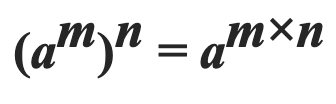#DAY 18
0
Software Development

## Function type - Another Algebraic Data Type

``````// Sum type：只有 Some 或是 None 兩種 ( T + 1 )
sealed class Maybe<T> {
class Some(value T): Maybe<T>
class None(); Maybe<T>
}

// Product type :  first, second 彼此是獨立的互不干擾，可能性為 (T * R)
class Pair<T, R>(val first: T, val second: R)

Maybe<Boolean> => 2 + 1 種可能的值
Pair<Boolean, Byte> => 2 * 256 種可能的值
``````

``````// 紅燈要停還是要走呢？不同地區應該有不同的策略ＸＤ
typealias Walk = (Light) -> Boolean

enum class Light { GREEN, YELLOW, RED}
// 不管是什麼燈，往前衝就對了
val walk1: (Light) -> Boolean = { light ->
when(light) {
Light.RED -> true
Light.GREEN -> true
Light.YELLOW -> true
}
}

val walk2: (Light) -> Boolean = { light ->
when(light) {
Light.RED -> true
Light.GREEN -> true
Light.YELLOW -> false
}
}

val walk3: (Light) -> Boolean = { light ->
when(light) {
Light.RED -> true
Light.GREEN -> false
Light.YELLOW -> true
}
}

val walk4: (Light) -> Boolean = { light ->
when(light) {
Light.RED -> true
Light.GREEN -> false
Light.YELLOW -> false
}
}

val walk5: (Light) -> Boolean = { light ->
when(light) {
Light.RED -> false
Light.GREEN -> true
Light.YELLOW -> true
}
}

val walk6: (Light) -> Boolean = { light ->
when(light) {
Light.RED -> false
Light.GREEN -> true
Light.YELLOW -> false
}
}

val walk7: (Light) -> Boolean = { light ->
when(light) {
Light.RED -> false
Light.GREEN -> false
Light.YELLOW -> true
}
}

val walk8: (Light) -> Boolean = { light ->
when(light) {
Light.RED -> false
Light.GREEN -> false
Light.YELLOW -> false
}
}
``````

``````function type: (T) -> R
number of possibilities: (number of R) ^ (number of T)
``````

# 更多的代數

``````a ^ 1 = a    //1
1 ^ a = 1    //2
``````
1. 任何數字的 1 次方還是等於本身的數字，還記得 1 可以替換成誰嗎？是 Unit ! ，所以等式的左邊可以替換成： `(Unit) → A` ，這是什麼函式呢？一個輸入是 Unit 的 function 幾乎等於沒有輸入只有輸出！也就是說可以把它看成 `getter()` ，所以總共是 A 種可能性，跟等式的右邊一樣。
2. 數字 1 的任何次方，就是等於 1 乘了很多次，那最後的結果當然還是等於 1。接著，等式的左邊 `1 ^ a` 可以替換成 `(A) → Unit` ，這又是什麼意思呢？ 這是一個完全不管輸入的 function ，輸出永遠是 Unit ， `fun boo(input: A) = Unit` ，所以只有一種可能性，與等式的右邊也相同。``````Left: (N) -> (M) -> A
Right: (Pair<N, M>) -> A
``````

``````fun left(n: N): (M) -> A { ... }
``````

Left 變成了我們比較熟悉的 Higher order function 了，返回值是一個 function type ，恩...？等等，在兩天前好像看過類似的結構不是嗎？ `left` 的返回類型可以轉為 Reader Monad ！

``````fun left(n: N): Reader<M, A> = { ... }
``````

``````fun right(pair: Pair<N, M>): A { ...}
``````

``````fun right(n: N, m: M): A {...}
// 一起拿來比較
fun left(n: N): Reader<M, A> = { ... }
``````

``````left = (Pair<N, M>) -> A = (N, M) -> A
right = (N) -> (M) -> A

(N, M) -> A = (N) -> (M) -> A
``````